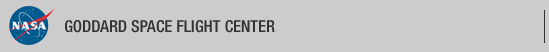+ Visit NASA.gov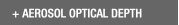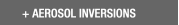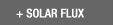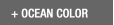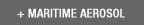Web Site Feature AERONET Data Synergy Tool - Access Earth Science data sets for AERONET sites+Home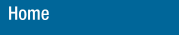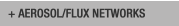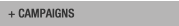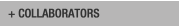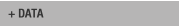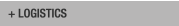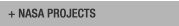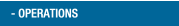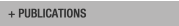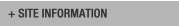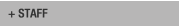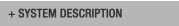AERONET DATA ACCESS

AERONET Site Lists (V3)

+ All Lists# Report of GSFC DCP transmissions

 Status Legend

AERONET No 6 [1317E33A] - 25 msg rcvd (12058 b) site : GSFC (Last Tx is before schedule by 0 min 3 sec
25 messages stepped outside their assigned time window)
AERONET No 26 [1316E1C0] - 25 msg rcvd (16828 b) site : GSFC (Last Tx is precisely on time)
AERONET No 134  - 23 msg rcvd (24878 b) site : GSFC (Last Tx is before schedule by 0 min 2 sec
23 messages stepped outside their assigned time window , 1b)
AERONET No 330 [131481D2] - 25 msg rcvd (22418 b) site : GSFC (Last Tx is precisely on time)

# Aeronet No 6 [1317E33A]

Last Tx is before schedule by 0 min 3 sec
25 messages stepped outside their assigned time window

12:12:2019,12:41:47 (UTC) 'G' (DCP total: 87) Cimel : 6 time corr. = -28 sec (DCP Volt = 13.00 Lat= 38.993 Long= -76.840(0.1 km from GSFC))
12:12:2019,13:41:47 (UTC) 'G' (DCP total: 673) Cimel : 6 time corr. = -29 sec (DCP Volt = 13.00 Lat= 38.993 Long= -76.840(0.0 km from GSFC)) staD nsu staD nsu staD nsu staD nsu staD nsu
12:12:2019,22:41:47 (UTC) 'G' (DCP total: 777) Cimel : 6 time corr. = -29 sec (DCP Volt = 13.00 Lat= 38.993 Long= -76.840(0.1 km from GSFC)) nsu staD nsu staD nsu staD nsu staD nsu staD nsu staD
12:12:2019,23:41:47 (UTC) 'G' (DCP total: 87) Cimel : 6 time corr. = -28 sec (DCP Volt = 13.00 Lat= 38.993 Long= -76.840(0.1 km from GSFC))
13:12:2019,00:41:47 (UTC) 'G' (DCP total: 87) Cimel : 6 time corr. = -28 sec (DCP Volt = 13.00 Lat= 38.993 Long= -76.840(0.0 km from GSFC))
13:12:2019,01:41:47 (UTC) 'G' (DCP total: 87) Cimel : 6 time corr. = -30 sec (DCP Volt = 13.00 Lat= 38.993 Long= -76.840(0.0 km from GSFC))
13:12:2019,02:41:47 (UTC) 'G' (DCP total: 87) Cimel : 6 time corr. = -30 sec (DCP Volt = 13.00 Lat= 38.993 Long= -76.840(0.0 km from GSFC))
13:12:2019,03:41:47 (UTC) 'G' (DCP total: 87) Cimel : 6 time corr. = -29 sec (DCP Volt = 13.00 Lat= 38.992 Long= -76.840(0.0 km from GSFC))
13:12:2019,04:41:47 (UTC) 'G' (DCP total: 87) Cimel : 6 time corr. = -30 sec (DCP Volt = 13.00 Lat= 38.993 Long= -76.840(0.0 km from GSFC))
13:12:2019,05:41:47 (UTC) 'G' (DCP total: 87) Cimel : 6 time corr. = -30 sec (DCP Volt = 13.00 Lat= 38.993 Long= -76.841(0.1 km from GSFC))
13:12:2019,06:41:47 (UTC) 'G' (DCP total: 87) Cimel : 6 time corr. = -29 sec (DCP Volt = 13.00 Lat= 38.992 Long= -76.840(0.0 km from GSFC))
13:12:2019,07:41:47 (UTC) 'G' (DCP total: 87) Cimel : 6 time corr. = -30 sec (DCP Volt = 13.00 Lat= 38.993 Long= -76.840(0.0 km from GSFC))
13:12:2019,08:41:47 (UTC) 'G' (DCP total: 87) Cimel : 6 time corr. = -30 sec (DCP Volt = 13.00 Lat= 38.993 Long= -76.840(0.0 km from GSFC))
13:12:2019,09:41:47 (UTC) 'G' (DCP total: 87) Cimel : 6 time corr. = -29 sec (DCP Volt = 13.00 Lat= 38.993 Long= -76.840(0.0 km from GSFC))
13:12:2019,10:41:47 (UTC) 'G' (DCP total: 87) Cimel : 6 time corr. = -30 sec (DCP Volt = 13.00 Lat= 38.994 Long= -76.840(0.1 km from GSFC))
13:12:2019,11:41:47 (UTC) 'G' (DCP total: 87) Cimel : 6 time corr. = -30 sec (DCP Volt = 13.00 Lat= 38.994 Long= -76.840(0.1 km from GSFC))
13:12:2019,12:41:47 (UTC) 'G' (DCP total: 87) Cimel : 6 time corr. = -29 sec (DCP Volt = 13.00 Lat= 38.993 Long= -76.841(0.1 km from GSFC))

# Aeronet No 26 [1316E1C0]

Last Tx is precisely on time

12:12:2019,12:32:41 (UTC) 'G' (DCP total: 89) Cimel : 26 time corr. = -3 sec (DCP Volt = 12.80 Lat= 38.992 Long= -76.840(0.0 km from GSFC))
12:12:2019,13:32:41 (UTC) 'G' (DCP total: 605) Cimel : 26 time corr. = -3 sec (DCP Volt = 12.80 Lat= 38.993 Long= -76.840(0.0 km from GSFC)) staA ssk staA ssk staA ssk staA
12:12:2019,14:32:41 (UTC) 'G' (DCP total: 1216) Cimel : 26 time corr. = -4 sec (DCP Volt = 14.20 Lat= 38.993 Long= -76.840(0.0 km from GSFC)) ssk staA ssk staA ssk staB ssk all(1) alr(1) all(2) alr(2) all(3) alr(3) all(4)
12:12:2019,15:32:41 (UTC) 'G' (DCP total: 1213) Cimel : 26 time corr. = -3 sec (DCP Volt = 14.10 Lat= 38.993 Long= -76.840(0.0 km from GSFC)) alr(4) blk staF nsu all(1) alr(1) all(2) alr(2) all(3) alr(3) all(4) alr(4) nsu pp1(1)
12:12:2019,16:32:41 (UTC) 'G' (DCP total: 1215) Cimel : 26 time corr. = -3 sec (DCP Volt = 14.00 Lat= 38.993 Long= -76.840(0.0 km from GSFC)) pp1(2) pp1(3) pp1(4) blk staD nsu staD nsu staD nsu staF nsu all(1) alr(1) all(2) alr(2)
12:12:2019,17:32:41 (UTC) 'G' (DCP total: 1213) Cimel : 26 time corr. = -4 sec (DCP Volt = 13.80 Lat= 38.993 Long= -76.840(0.0 km from GSFC)) all(3) alr(3) all(4) alr(4) nsu pp1(1) pp1(2) pp1(3) pp1(4) blk staD nsu staD nsu
12:12:2019,18:32:41 (UTC) 'G' (DCP total: 1215) Cimel : 26 time corr. = -3 sec (DCP Volt = 13.70 Lat= 38.993 Long= -76.840(0.0 km from GSFC)) staD nsu staF nsu all(1) alr(1) all(2) alr(2) all(3) alr(3) all(4) alr(4) nsu pp1(1)
12:12:2019,19:32:41 (UTC) 'G' (DCP total: 1214) Cimel : 26 time corr. = -3 sec (DCP Volt = 13.60 Lat= 38.993 Long= -76.840(0.0 km from GSFC)) pp1(2) pp1(3) pp1(4) blk staD nsu staD nsu staD nsu staF nsu all(1) alr(1) all(2)
12:12:2019,20:32:41 (UTC) 'G' (DCP total: 1213) Cimel : 26 time corr. = -4 sec (DCP Volt = 13.50 Lat= 38.992 Long= -76.840(0.0 km from GSFC)) alr(2) all(3) alr(3) all(4) alr(4) nsu pp1(1) pp1(2) pp1(3) pp1(4) blk staD nsu staD
12:12:2019,21:32:41 (UTC) 'G' (DCP total: 1216) Cimel : 26 time corr. = -3 sec (DCP Volt = 13.40 Lat= 38.993 Long= -76.840(0.0 km from GSFC)) nsu staD nsu staF nsu all(1) alr(1) all(2) alr(2) all(3) alr(3) all(4) alr(4) nsu
12:12:2019,22:32:41 (UTC) 'G' (DCP total: 1214) Cimel : 26 time corr. = -3 sec (DCP Volt = 13.10 Lat= 38.993 Long= -76.840(0.0 km from GSFC)) pp1(1) pp1(2) pp1(3) pp1(4) blk staD nsu staD nsu staD nsu staF nsu all(1) alr(1) all(2)
12:12:2019,23:32:41 (UTC) 'G' (DCP total: 1213) Cimel : 26 time corr. = -4 sec (DCP Volt = 13.10 Lat= 38.993 Long= -76.840(0.0 km from GSFC)) alr(2) all(3) alr(3) all(4) alr(4) nsu pp1(1) pp1(2) pp1(3) pp1(4) blk staD nsu
13:12:2019,00:32:41 (UTC) 'G' (DCP total: 1216) Cimel : 26 time corr. = -3 sec (DCP Volt = 13.00 Lat= 38.993 Long= -76.840(0.0 km from GSFC)) staD nsu staD nsu staF nsu all(1) alr(1) all(2) alr(2) all(3) alr(3) all(4) alr(4) nsu
13:12:2019,01:32:41 (UTC) 'G' (DCP total: 1213) Cimel : 26 time corr. = -3 sec (DCP Volt = 13.00 Lat= 38.993 Long= -76.840(0.0 km from GSFC)) pp1(1) pp1(2) pp1(3) pp1(4) blk staB ssk all(1) alr(1) all(2) alr(2) all(3)
13:12:2019,02:32:41 (UTC) 'G' (DCP total: 1123) Cimel : 26 time corr. = -4 sec (DCP Volt = 13.00 Lat= 38.993 Long= -76.840(0.0 km from GSFC)) alr(3) all(4) alr(4) blk staA ssk staA ssk staA ssk staA ssk staA ssk staA
13:12:2019,03:32:41 (UTC) 'G' (DCP total: 89) Cimel : 26 time corr. = -3 sec (DCP Volt = 13.00 Lat= 38.993 Long= -76.840(0.0 km from GSFC))
13:12:2019,04:32:41 (UTC) 'G' (DCP total: 89) Cimel : 26 time corr. = -3 sec (DCP Volt = 13.00 Lat= 38.993 Long= -76.840(0.0 km from GSFC))
13:12:2019,05:32:41 (UTC) 'G' (DCP total: 89) Cimel : 26 time corr. = -4 sec (DCP Volt = 12.90 Lat= 38.993 Long= -76.840(0.0 km from GSFC))
13:12:2019,06:32:41 (UTC) 'G' (DCP total: 89) Cimel : 26 time corr. = -3 sec (DCP Volt = 12.90 Lat= 38.992 Long= -76.840(0.0 km from GSFC))
13:12:2019,07:32:41 (UTC) 'G' (DCP total: 89) Cimel : 26 time corr. = -3 sec (DCP Volt = 12.90 Lat= 38.993 Long= -76.840(0.0 km from GSFC))
13:12:2019,08:32:41 (UTC) 'G' (DCP total: 89) Cimel : 26 time corr. = -4 sec (DCP Volt = 12.80 Lat= 38.993 Long= -76.840(0.0 km from GSFC))
13:12:2019,09:32:41 (UTC) 'G' (DCP total: 89) Cimel : 26 time corr. = -3 sec (DCP Volt = 12.80 Lat= 38.993 Long= -76.840(0.0 km from GSFC))
13:12:2019,10:32:41 (UTC) 'G' (DCP total: 89) Cimel : 26 time corr. = -3 sec (DCP Volt = 12.80 Lat= 38.992 Long= -76.840(0.0 km from GSFC))
13:12:2019,11:32:41 (UTC) 'G' (DCP total: 89) Cimel : 26 time corr. = -4 sec (DCP Volt = 12.80 Lat= 38.993 Long= -76.840(0.0 km from GSFC))
13:12:2019,12:32:41 (UTC) 'G' (DCP total: 89) Cimel : 26 time corr. = -3 sec (DCP Volt = 12.80 Lat= 38.993 Long= -76.840(0.0 km from GSFC))

# Aeronet No 134 

Last Tx is before schedule by 0 min 2 sec
23 messages stepped outside their assigned time window

12:12:2019,14:30:38 (UTC) Message NO DATA
12:12:2019,15:30:38 (UTC) 'G' (DCP total: 1212) Cimel : 134 time corr. = 9 sec (DCP Volt = 12.30 Lat= 38.993 Long= -76.840(0.0 km from GSFC)) pp1(2) pp1(3) pp1(4) blk staD nsu staD nsu staD nsu staF nsu all(1) alr(1)
12:12:2019,16:30:38 (UTC) 'G' (DCP total: 1212) Cimel : 134 time corr. = 9 sec (DCP Volt = 12.30 Lat= 38.993 Long= -76.840(0.0 km from GSFC)) all(2) alr(2) all(3) alr(3) all(4) alr(4) nsu pp1(1) pp1(2) pp1(3) pp1(4) blk staD
12:12:2019,17:30:38 (UTC) 'G' (DCP total: 1215) Cimel : 134 time corr. = 9 sec (DCP Volt = 12.50 Lat= 38.993 Long= -76.840(0.0 km from GSFC)) nsu staD nsu staD nsu staF nsu all(1) alr(1) all(2) alr(2) all(3) alr(3) all(4) alr(4)
12:12:2019,18:30:38 (UTC) 'G' (DCP total: 1213) Cimel : 134 time corr. = 9 sec (DCP Volt = 12.70 Lat= 38.992 Long= -76.840(0.0 km from GSFC)) nsu pp1(1) pp1(2) pp1(3) pp1(4) blk staD nsu staD nsu staD nsu staF nsu all(1) alr(1)
12:12:2019,19:30:38 (UTC) 'G' (DCP total: 1213) Cimel : 134 time corr. = 9 sec (DCP Volt = 12.70 Lat= 38.993 Long= -76.839(0.1 km from GSFC)) all(2) alr(2) all(3) alr(3) all(4) alr(4) nsu pp1(1) pp1(2) pp1(3) pp1(4)
12:12:2019,20:30:38 (UTC) 'G' (DCP total: 1214) Cimel : 134 time corr. = 9 sec (DCP Volt = 12.60 Lat= 38.993 Long= -76.840(0.0 km from GSFC)) blk all(3) alr(3) all(4) alr(4) blk staA ssk staA ssk staA ssk staA ssk staA ssk staA
12:12:2019,21:30:38 (UTC) 'G' (DCP total: 1213) Cimel : 134 time corr. = 9 sec (DCP Volt = 12.50 Lat= 38.992 Long= -76.840(0.0 km from GSFC)) ssk staA ssk staA ssk staA ssk staA ssk staA ssk staA ssk staB ssk all(1)
12:12:2019,22:30:38 (UTC) 'G' (DCP total: 1214) Cimel : 134 time corr. = 9 sec (DCP Volt = 12.30 Lat= 38.993 Long= -76.840(0.1 km from GSFC)) alr(1) all(2) alr(2) all(3) alr(3) all(4) alr(4) blk staF nsu all(1) alr(1) all(2) alr(2)
12:12:2019,23:30:38 (UTC) 'G' (DCP total: 1212) Cimel : 134 time corr. = 9 sec (DCP Volt = 12.10 Lat= 38.993 Long= -76.840(0.1 km from GSFC)) all(3) alr(3) all(4) alr(4) nsu stab staD nsu staD nsu staD nsu staF nsu all(1) alr(1) all(2)
13:12:2019,00:30:38 (UTC) 'G' (DCP total: 1212) Cimel : 134 time corr. = 9 sec (DCP Volt = 12.00 Lat= 38.992 Long= -76.840(0.0 km from GSFC)) alr(2) all(3) alr(3) all(4) alr(4) nsu pp1(1) pp1(2) pp1(3) pp1(4) blk staD nsu
13:12:2019,01:30:38 (UTC) 'G' (DCP total: 1215) Cimel : 134 time corr. = 9 sec (DCP Volt = 11.90 Lat= 38.993 Long= -76.840(0.0 km from GSFC)) staD nsu staD nsu staF nsu all(1) alr(1) all(2) alr(2) all(3) alr(3) all(4) alr(4) nsu
13:12:2019,02:30:38 (UTC) 'G' (DCP total: 1212) Cimel : 134 time corr. = 9 sec (DCP Volt = 11.90 Lat= 38.993 Long= -76.840(0.0 km from GSFC)) pp1(1) pp1(2) pp1(3) pp1(4) blk staD nsu staD nsu staD nsu staF nsu all(1) alr(1)
13:12:2019,03:30:38 (UTC) 'G' (DCP total: 1212) Cimel : 134 time corr. = 9 sec (DCP Volt = 11.90 Lat= 38.993 Long= -76.840(0.0 km from GSFC)) all(2) alr(2) all(3) alr(3) all(4) alr(4) nsu pp1(1) pp1(2) pp1(3) pp1(4) blk staD
13:12:2019,04:30:38 (UTC) 'G' (DCP total: 1215) Cimel : 134 time corr. = 9 sec (DCP Volt = 11.80 Lat= 38.993 Long= -76.840(0.0 km from GSFC)) nsu staD nsu staD nsu staF nsu all(1) alr(1) all(2) alr(2) all(3) alr(3) all(4) alr(4)
13:12:2019,05:30:38 (UTC) 'G' (DCP total: 1213) Cimel : 134 time corr. = 9 sec (DCP Volt = 11.80 Lat= 38.993 Long= -76.840(0.0 km from GSFC)) nsu pp1(1) pp1(2) pp1(3) pp1(4) blk staD nsu staD nsu staD nsu staF nsu all(1) alr(1)
13:12:2019,06:30:38 (UTC) 'G' (DCP total: 1212) Cimel : 134 time corr. = 9 sec (DCP Volt = 11.80 Lat= 38.993 Long= -76.840(0.0 km from GSFC)) all(2) alr(2) all(3) alr(3) all(4) alr(4) nsu pp1(1) pp1(2) pp1(3) pp1(4) blk
13:12:2019,07:30:38 (UTC) 'G' (DCP total: 1215) Cimel : 134 time corr. = 9 sec (DCP Volt = 11.80 Lat= 38.993 Long= -76.840(0.0 km from GSFC)) staD nsu staD nsu staD nsu staF nsu all(1) alr(1) all(2) alr(2) all(3) alr(3) all(4) alr(4)
13:12:2019,08:30:38 (UTC) 'G' (DCP total: 1212) Cimel : 134 time corr. = 9 sec (DCP Volt = 11.80 Lat= 38.993 Long= -76.840(0.0 km from GSFC)) nsu pp1(1) pp1(2) pp1(3) pp1(4) blk staB ssk all(1) alr(1) all(2) alr(2)
13:12:2019,09:30:38 (UTC) 'G' (DCP total: 1211) Cimel : 134 time corr. = 9 sec (DCP Volt = 11.80 Lat= 38.993 Long= -76.840(0.0 km from GSFC)) all(3) alr(3) all(4) alr(4) blk staA ssk staA ssk staA ssk staA ssk staA ssk staA
13:12:2019,10:30:38 (UTC) 'G' (DCP total: 88) Cimel : 134 time corr. = 9 sec (DCP Volt = 11.80 Lat= 38.993 Long= -76.840(0.1 km from GSFC))
13:12:2019,11:30:38 (UTC) 'G' (DCP total: 88) Cimel : 134 time corr. = 9 sec (DCP Volt = 11.80 Lat= 38.993 Long= -76.840(0.1 km from GSFC))
13:12:2019,12:30:38 (UTC) 'G' (DCP total: 88) Cimel : 134 time corr. = 9 sec (DCP Volt = 11.70 Lat= 38.993 Long= -76.840(0.0 km from GSFC))

# Aeronet No 330 [131481D2]

Last Tx is precisely on time

12:12:2019,12:38:01 (UTC) 'G' (DCP total: 87) Cimel : 330 time corr. = -70 sec (DCP Volt = 13.30 Lat= 38.993 Long= -76.840(0.0 km from GSFC))
12:12:2019,13:38:01 (UTC) 'G' (DCP total: 692) Cimel : 330 time corr. = -70 sec (DCP Volt = 13.30 Lat= 38.993 Long= -76.840(0.0 km from GSFC)) staA nsu staA nsu staA nsu staA nsu staA
12:12:2019,14:38:01 (UTC) 'G' (DCP total: 1211) Cimel : 330 time corr. = -70 sec (DCP Volt = 13.30 Lat= 38.993 Long= -76.840(0.0 km from GSFC)) nsu staA nsu staB nsu all(1) alr(1) all(21) alr(21) all(4) alr(4) all(24) alr(24) all(5)
12:12:2019,15:38:01 (UTC) 'G' (DCP total: 1211) Cimel : 330 time corr. = -70 sec (DCP Volt = 13.30 Lat= 38.993 Long= -76.840(0.0 km from GSFC)) alr(5) all(19) alr(19) staF nsu all(1) alr(1) all(21) alr(21) all(4) alr(4) all(24) alr(24)
12:12:2019,16:38:01 (UTC) 'G' (DCP total: 1211) Cimel : 330 time corr. = -70 sec (DCP Volt = 13.30 Lat= 38.993 Long= -76.840(0.0 km from GSFC)) all(5) alr(5) all(19) alr(19) nsu pp1(1) pp1(21) pp1(4) pp1(24) pp1(5)
12:12:2019,17:38:01 (UTC) 'G' (DCP total: 1213) Cimel : 330 time corr. = -71 sec (DCP Volt = 13.30 Lat= 38.993 Long= -76.840(0.0 km from GSFC)) pp1(19) blk staD nsu staD nsu staD nsu staF nsu all(1) alr(1) all(21) alr(21) all(4) alr(4)
12:12:2019,18:38:01 (UTC) 'G' (DCP total: 1213) Cimel : 330 time corr. = -70 sec (DCP Volt = 13.30 Lat= 38.993 Long= -76.840(0.0 km from GSFC)) all(24) alr(24) all(5) alr(5) all(19) alr(19) nsu pp1(1) pp1(21) pp1(4) pp1(24)
12:12:2019,19:38:01 (UTC) 'G' (DCP total: 1211) Cimel : 330 time corr. = -70 sec (DCP Volt = 13.30 Lat= 38.993 Long= -76.839(0.1 km from GSFC)) pp1(5) pp1(19) blk staD nsu staD nsu staD nsu staF nsu all(1) alr(1) all(21) alr(21)
12:12:2019,20:38:01 (UTC) 'G' (DCP total: 1211) Cimel : 330 time corr. = -71 sec (DCP Volt = 13.30 Lat= 38.993 Long= -76.840(0.0 km from GSFC)) all(4) alr(4) all(24) alr(24) all(5) alr(5) all(19) alr(19) nsu pp1(1) pp1(21)
12:12:2019,21:38:01 (UTC) 'G' (DCP total: 1213) Cimel : 330 time corr. = -70 sec (DCP Volt = 13.30 Lat= 38.993 Long= -76.840(0.0 km from GSFC)) pp1(4) pp1(24) pp1(5) pp1(19) blk staD nsu staD nsu staD nsu staF nsu all(1) alr(1)
12:12:2019,22:38:01 (UTC) 'G' (DCP total: 1213) Cimel : 330 time corr. = -70 sec (DCP Volt = 13.30 Lat= 38.993 Long= -76.840(0.1 km from GSFC)) all(21) alr(21) all(4) alr(4) all(24) alr(24) all(5) alr(5) all(19) alr(19) nsu
12:12:2019,23:38:01 (UTC) 'G' (DCP total: 1211) Cimel : 330 time corr. = -72 sec (DCP Volt = 13.30 Lat= 38.993 Long= -76.840(0.1 km from GSFC)) pp1(1) pp1(21) pp1(4) pp1(24) pp1(5) pp1(19) blk staD nsu staD nsu staD nsu staF nsu
13:12:2019,00:38:01 (UTC) 'G' (DCP total: 1214) Cimel : 330 time corr. = -72 sec (DCP Volt = 13.30 Lat= 38.992 Long= -76.840(0.0 km from GSFC)) all(1) alr(1) all(21) alr(21) all(4) alr(4) all(24) alr(24) all(5) alr(5) all(19) alr(19)
13:12:2019,01:38:01 (UTC) 'G' (DCP total: 1214) Cimel : 330 time corr. = -71 sec (DCP Volt = 13.30 Lat= 38.992 Long= -76.840(0.0 km from GSFC)) nsu pp1(1) pp1(21) pp1(4) pp1(24) pp1(5) pp1(19) blk staD nsu staD nsu staD
13:12:2019,02:38:01 (UTC) 'G' (DCP total: 1211) Cimel : 330 time corr. = -72 sec (DCP Volt = 13.30 Lat= 38.993 Long= -76.840(0.0 km from GSFC)) nsu staF nsu all(1) alr(1) all(21) alr(21) all(4) alr(4) all(24) alr(24) all(5) alr(5)
13:12:2019,03:38:01 (UTC) 'G' (DCP total: 1213) Cimel : 330 time corr. = -72 sec (DCP Volt = 13.30 Lat= 38.993 Long= -76.840(0.0 km from GSFC)) all(19) alr(19) nsu pp1(1) pp1(21) pp1(4) pp1(24) pp1(5) pp1(19) blk staD
13:12:2019,04:38:01 (UTC) 'G' (DCP total: 1211) Cimel : 330 time corr. = -71 sec (DCP Volt = 13.30 Lat= 38.993 Long= -76.840(0.0 km from GSFC)) nsu staD nsu staD nsu staF nsu all(1) alr(1) all(21) alr(21) all(4) alr(4) all(24) alr(24)
13:12:2019,05:38:01 (UTC) 'G' (DCP total: 1211) Cimel : 330 time corr. = -72 sec (DCP Volt = 13.30 Lat= 38.993 Long= -76.840(0.0 km from GSFC)) all(5) alr(5) all(19) alr(19) nsu pp1(1) pp1(21) pp1(4) pp1(24) pp1(5)
13:12:2019,06:38:01 (UTC) 'G' (DCP total: 1213) Cimel : 330 time corr. = -72 sec (DCP Volt = 13.30 Lat= 38.992 Long= -76.840(0.0 km from GSFC)) pp1(19) blk staB nsu all(1) alr(1) all(21) alr(21) all(4) alr(4) all(24) alr(24) all(5)
13:12:2019,07:38:01 (UTC) 'G' (DCP total: 999) Cimel : 330 time corr. = -72 sec (DCP Volt = 13.30 Lat= 38.993 Long= -76.840(0.0 km from GSFC)) alr(5) all(19) alr(19) staA nsu staA nsu staA nsu staA nsu staA nsu staA
13:12:2019,08:38:01 (UTC) 'G' (DCP total: 87) Cimel : 330 time corr. = -72 sec (DCP Volt = 13.30 Lat= 38.993 Long= -76.840(0.0 km from GSFC))
13:12:2019,09:38:01 (UTC) 'G' (DCP total: 87) Cimel : 330 time corr. = -72 sec (DCP Volt = 13.30 Lat= 38.992 Long= -76.840(0.0 km from GSFC))
13:12:2019,10:38:01 (UTC) 'G' (DCP total: 87) Cimel : 330 time corr. = -72 sec (DCP Volt = 13.30 Lat= 38.994 Long= -76.840(0.1 km from GSFC))
13:12:2019,11:38:01 (UTC) 'G' (DCP total: 87) Cimel : 330 time corr. = -72 sec (DCP Volt = 13.30 Lat= 38.994 Long= -76.840(0.1 km from GSFC))
13:12:2019,12:38:01 (UTC) 'G' (DCP total: 87) Cimel : 330 time corr. = -74 sec (DCP Volt = 13.30 Lat= 38.993 Long= -76.840(0.0 km from GSFC))+ Privacy Policy and Important Notices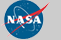Curator: David M. Giles NASA Official: Brent N. Holben Generated: 13/12/2019